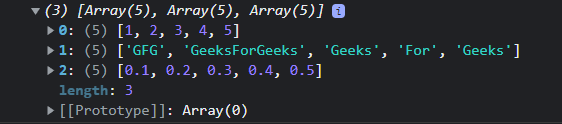GeeksforGeeks App
Open AppBrowser
Continue

# How to create an array of elements, ungrouping the elements in an array produced by zip in JavaScript ?

We can create an array of elements by ungrouping the elements in an array produced by zip by using different methods in JavaScript such as Math.max(), array specific methods namely Array.prototype.map(), Array.prototype.reduce() and Array.prototype.forEach(). This operation can also be termed as unzip.

Before looking at unzip, let us understand what zip does. Zip creates an array of elements, grouped based on their position in the original arrays. To achieve this, we can make use of JavaScript methods such as Math.max(), Array.from(), and Array.prototype.map()

Approach:

• Math.max(): To get the longest array out of all argument arrays.
• Then, create an array with that length as a return value and use Array.from() with a mapping function to create an array of grouped elements.
• Array.prototype.map(): To convert every array element into a new array.
• Moreover, the spread operator (…) is used to represent a variable number of arrays that can be passed as arguments in the parameter field of the zip function.

Example: This example demonstrates the above-explained approach.

## Javascript

 ``

Output:Now let us look at how we can implement unzip:

Example: This example demonstrates the above-explained approach.

## Javascript

 ``

Output:

`[3,6,18]`

My Personal Notes arrow_drop_up